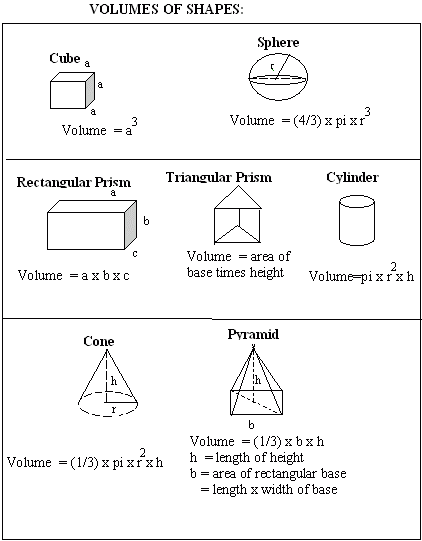Email us to get an instant 20% discount on highly effective K-12 Math & English kwizNET Programs!

#### Online Quiz (WorksheetABCD)

Questions Per Quiz = 2 4 6 8 10

### MEAP Preparation - Grade 6 Mathematics3.34 Volumes of Figures

 Volume is a measure of how much space a space figure takes up. Volume is used to measure a space figure just as area is used to measure a plane figure. Directions: Review the following table and answer the questions.Examples: The volume of a cube with side length 4 is 4 x 4 x 4 = 64. The volume of a rectangular prism with length 5, width 4 and height 2 is 5 x 4 x 2 = 40. The volume of a cylinder with radius 2 and height 4 is pi x 2 x 2 x 4 = 16 pi. The volume of a triangular prism with area of triangular base 8 and height 5 is 8 x 5 = 40. The volume of a cone with radius 2 and height 9 is pi x 2 x 2 x 9 x 1/3 = 12 pi. The volume of a sphere of radius 6 is pi x 6 x 6 x 6 x 4/3 = 288 pi. Directions: Answer the following questions. Also write at least 2 examples to find the volume of each kind of figure.
 Q 1: The volume of a rectangular prism with length 2 cm, width 3 cm and height 5 cm is ___ cubic cm .Answer: Q 2: The volume of a cube with side length 2 cm is ____ cm3.Answer: Q 3: The volume of a sphere of radius 3 is _____ .30 pi36 pi18 pi Q 4: The volume of a cube with side length 3 cm is ____ cm3.Answer: Q 5: The volume of a cube with side 'a' is given byV = 3 x aV = a x a x aV = 3a Q 6: The volume of a cylinder with radius 3 and height 5 is ___. 45 pi15 pi50 pi Question 7: This question is available to subscribers only! Question 8: This question is available to subscribers only!